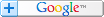設為首頁加入最愛加入會員會員登入玩 友 天 地 刊登廣告 冰涼薄荷糖: 各種埋藏黑歷史的地方 卡爾: 119687) 定期會回來看看，入群嗎兄弟 Moriarty: 天尊王卡爾 還在啊 珍珠奶茶專賣店 » 賣了24天研究出不錯的配方~ 粉圓:24公克 茶葉:7.47公克 奶精:29.3公克 糖:3... 樂高殭屍屋: 雙人殺戮 » 好久都沒出新遊戲了 這地方是不是荒廢了 混沌格鬥 » 好玩!!!!!!搜尋遊戲名稱» 打開我的口袋 » 加入我的最愛 » 我要推薦遊戲 » 看我的居落格 » 看我的留言板

 » 首頁遊戲 » 新進遊戲 » 發燒排行 » 票選排行 » 隨機播放 »輕鬆小品抓狂關卡誰去買珍奶恐怖血腥多人對戰清涼養眼動作射擊冒險解謎角色扮演格鬥運動戰略賽車飛行經營養成作菜點心懷舊經典方塊益智紙牌桌上戀愛音樂換衣服怪怪魔塔 51層遊戲類型: 角色扮演,方塊益智 無神月3322 次 101344 次 2.29 (滿分5.00) 投票數: 792 選擇評分 5 - 超完美 4 - 好好玩 3 - 還可以 2 - 很勉強 1 - 遜掉了

21層魔塔可在這裏玩得到: http://mygamespocket.com/game_detail.php?game_index=669

[←→↑↓] 上下左右移動，移動到怪物格，就會和怪物一決生死
[空白鍵] 打開遊戲說明，全中文的喔
[S] 遊戲存檔
[L] 載入存檔
[R] 遊戲重新開始(小心不要按錯)
[H] 在第三層從老人身上拿到寶物後，可查看目前樓層的所有怪物屬性
[文編:Haska Su/我的遊戲口袋]

 魔塔 51層: 本遊戲版權歸原作者所有，如果無意中侵犯了您的版權，請來信告知，本站將於三日內儘速處理。 [SOURCE: http://sohippy.org]方塊養成 : GROW系列的變化版-正方體。 按你喜愛的順序點選周圍的小物品，讓小方塊一步一步成長。不一樣的順序會長出不一樣的世界喔! 試著讓所有的東...調酒大師 : 想嘗嘗在高級吧台當調酒師嗎? 這款小遊戲可以讓你盡情發揮想像力，調配出你心目中各種好喝的雞尾酒… 當然嘍，也可以調出讓人吐血的噁心酒啦! ...魔塔 : 超經典的迷宮RPG! 除了享受古典RPG的樂趣，還要動腦筋想出過關的順序，才能順利救出公主喔! 闖入神奇的《魔塔》遊戲的劇情並不複雜，讓玩家去...轟炸機器人 : 令人懷念的轟炸超人(彈水阿給)，現在有網頁版小遊戲可以和朋友互相撕殺嘍! 放炸彈、搶寶物，看誰先轟飛對手，誰就是贏家。遊戲可以選單人遊...66層魔塔 - 靈山聖戰 : 改篇自原祖魔塔小遊戲，玩家扮演孫悟空，要大鬧這個66層的佛塔。 故事: 故事發生在孫悟空成功護送唐三藏西天取經後，師徒四人一起回靈山接...遊戲討論區 : 共 62 篇 魔塔 51層
 62) 從心開始 [| 留言板 | 居落格 | 遊戲口袋 ] 2012-07-15 01:18:1464的弟21層怎過去阿 回覆本篇評論 | 閱讀網友意見(1) 61) 乂神秘使者乂 [| 留言板 | 居落格 | 遊戲口袋 ] 2011-06-19 03:28:06這魔塔太簡單了= =打怪不扣血反而加血= = 到44樓粉複雜= =反正 你要拿到炸彈 跟自動上(下)樓的特殊物品 先到43(如果是下樓要到45) 樓 使用你的特殊物品 到44樓後 用炸彈在守衛中 間炸死他們 因為你打不過他們 殺死後 就拿得到無敵盾牌(自創名)可防禦魔法~ 回覆本篇評論 | 閱讀網友意見(0) 60) Moriarty [| 留言板 | 居落格 | 遊戲口袋 ] 2010-01-10 10:35:43這遊戲有滿多版的 我比較喜歡拿到武器可以顯示出來 不要只有拿到就+攻擊力而已 顯示出來比較帥 盾牌也是 回覆本篇評論 | 閱讀網友意見(0) 59) linching [| 留言板 | 居落格 | 遊戲口袋 ] 2009-09-17 17:15:36 翻譯過的攻略:(我是在別ㄉ地方下載然後翻譯的) 攻略︰注︰L－生命，A－攻擊力，D－防禦力，G－金錢。 1－10層︰ 　　1-2F︰剛上來是按照預設劇情丟掉600血和兵器防具，行走路線只有一條，所以無話可說。 　　3F︰和小偷一起越獄之後，直接上3F，再上中吃完瓶子和鑰匙[L=1000，A=10，D=10，G=4]，向右打綠色史萊姆[L=976，G=5]，找老頭要手冊，然後向下打紅色史萊姆[L=926，G=7]，開門上樓。 　　4F︰過黃門，直接向左，打綠色史萊姆[L=902，G=8]，打紅色史萊姆[L=852，G=10]，開門上樓。 　　5F︰向上打綠色史萊姆[L=828，G=11]，向右打綠色史萊姆[L=804，G=12]，向下打紅色史萊姆[L=754，G=14]，開門拿劍[L=754，A=20，D=10，G=14]。向上打紅色史萊姆[L=734，G=16]，不過門，回到中路打蝙蝠拿鑰匙[L=678，G=19]，下樓。 　　4F︰直接過黃門打紅色史萊姆[L=658，G=21]，再過藍門去商店，升級防禦[L=658，A=20，D=14，G=1]。上樓。 　　5F︰到右上角取鑰匙，兩個綠色史萊姆只打一個[L=654]。到中路過門打紅色史萊姆[L=6 42]，打蝙蝠[L=594]，開門上樓。 　　6F︰向下右轉打紅色史萊姆[L=582]，打初級法師[L=510]，拿鑰匙，向上左轉，進門打紅色史萊姆[L=498]拿鑰匙，然後向下過兩個門，打紅色史萊姆[L=486]補血[L=586]，過門紅色史萊姆[L=574]，上樓。 　　7F︰向上出門，左轉走到初級法師面前向下，打蝙蝠拿鑰匙[L=526]，打初級法師[L=454]，打骷髏戰士[L=226]，開門上樓。 　　8F︰向下開門吃瓶[L=276]打紅/綠/紅色史萊姆[L=248]，向下開門右轉打骷髏[L=16 4]，向上打蝙蝠[L=116]，再向上打初級法師[L=44]，拿鑰匙開門上樓。 　　9F︰向右開門打綠色史萊姆[L=40]，吃瓶[L=90]，向下走到兩扇門前再向下，從漏洞取盾[L=90，A=20，D=24，G=56]，回頭一直下到5樓。 　　5F︰過門向下打蝙蝠[L=62]再回到9樓。 　　9F︰過兩個黃門拿鑰匙和紅寶石[L=62，A=21，D=24，G=59]，再向左過黃門，打蝙蝠[L=48]，拿鑰匙和藍寶石[L=48，A=21，D=25，G=62]，回頭一直下到7樓。 　　7F︰在上樓梯邊上那一豎列，開門，打蝙蝠[L=35]，吃瓶拿紅寶石[L=85，A=22，D=25，G=65]，然後一直下到3樓。 　　3F︰去左上角，打蝙蝠[L=72]，向上過門打初級法師[L=44]，拿鑰匙、藍寶石和血瓶[L=94，A=22，D=26，G=73]，然後上4樓。 　　4F︰去左上角，打初級法師[L=70]，吃血拿黃/藍鑰匙[L=120]，上8樓。 　　8F︰去左下角打蝙蝠[L=108]，過黃門打綠色史萊姆[不傷]，打蝙蝠[L=96]，過藍門拿鑰匙和紅/藍寶石[L=96，A=23，D=27，G=85]，下到1樓。 　　1F︰去右中附近，過黃門打蝙蝠[L=85]，打初級法師[L=70]，打蝙蝠[L=59]，進黃門拿鑰匙、血瓶和紅/藍寶石[L=109，A=24，D=28，G=96]，然後去4樓。 　　4F︰去左下過黃門，打蝙蝠[L=99]拿2把鑰匙，去6樓。 　　6F︰去左下打骷髏[L=71]，打綠色史萊姆[不傷]，過黃門打蝙蝠[L=61]，拿藍寶石並和老頭說話[L=61，A=24，D=29，G=109]，回頭下到3樓。 　　3F︰去左中過黃門打骷髏[L=35]，拿鑰匙、血瓶和紅寶石[L=85，A=25，D=29，G=115]，上到4樓。 　　4F︰下中偏右，過黃門打初級法師[L=76]，拿紅寶石和血瓶[L=126，A=26，D=29，G=120]，上到5樓。 　　5F︰左下方向打骷髏戰士[L=57]，拿鑰匙、藍寶石、血瓶和記事本[L=107，A=26，D=30，G=128]，然後下到4樓。 　　4F︰到左下打綠色史萊姆[不傷]，拿鑰匙，下到1樓。 　　1F︰左中方向過黃門吃瓶打骷髏[L=133]，向下過門拿鑰匙打骷髏戰士[L=67]，過黃門拿血瓶[L=167]、升降梯和鑰匙，然後上到7樓。 　　7F︰右數第2列完全打通，向上打骷髏[L=143]，拿血瓶和鑰匙[L=193]，向下打骷髏戰士[L=127]，拿血瓶和鑰匙[L=327]，然後上到9樓。 　　9F︰沿路向下中走，打紅色史萊姆[不傷]，打蝙蝠[L=319]，過黃門向左，向上打骷髏[L=295]，過黃門拿鑰匙打骷髏戰士[L=229]，過黃門拿鑰匙打骷髏戰士[L=163]，過黃門拿血瓶上樓。 　　10F︰過黃門打骷髏[L=189]，拿藍寶石[L=189，A=26，D=31，G=190]，向下過黃門打兩個初級法師[L=183]，過黃門打骷髏[L=161]，拿紅寶石[L=161，A=27，D=31，G=206]。到此為止，第一關升級完成，下面是補點血準備過關了。目前過關需要530的血[6個骷髏+2個骷髏戰士+骷髏隊長=6X22+2X63+272=530]。所以先去找血，下到9樓。 　　9F︰右下角過黃門打初級法師[L=158]，拿血瓶和鑰匙[L=208]，然後下到4樓。 　　4F︰去右上打骷髏[L=186]，過黃門打骷髏戰士[L=123]，拿血瓶和鑰匙[L=323]，和老頭說話，然後上到8樓。 　　8F︰右中偏下打初級法師[L=320]，過黃門打兩個初級衛兵[L=14]，拿血瓶和鑰匙[L=26 4]，然後下到7樓。 　　7F︰右數第3列下方，過黃門打紅色史萊姆[不傷]，打初級法師[L=261]，拿血瓶[L=461]，然後下到1樓。 　　1F︰右下角過黃門打蝙蝠[L=454]，拿血瓶[L=654]，然後上10樓。 　　10F︰決斗開始，一場屠殺之后勝利[L=124]，拿戰利品紅/藍寶石、鑰匙和1個血瓶[L=324，A=30，D=34，G=346]。 至此，第一關1-10層已經完成，但很多人都沒有殺，很多血瓶沒有拿，現列舉如下︰ 　　1F︰綠色史萊姆X2 　　2F︰老樣子，沒動 　　3F︰初級法師X1，蝙蝠X1； 　　紅瓶X2，黃鑰匙X1。 　　4F︰綠色史萊姆X2 　　5F︰初級法師X1，綠色史萊姆X1 　　6F︰骷髏戰士X1，骷髏X2，初級法師X2，蝙蝠X1，綠色史萊姆X1； 　　紅瓶X1，黃鑰匙X1。 　　7F︰骷髏戰士X1，紅色史萊姆X3，綠色史萊姆X4 　　8F︰骷髏戰士X1，骷髏X1，綠色史萊姆X1 　　藍鑰匙X1，黃鑰匙X1，紅瓶X1。 　　9F︰骷髏戰士X2，骷髏X2，初級法師X1，綠色史萊姆X1 　　黃鑰匙X1。 　　10F︰骷髏戰士X2，骷髏X2； 　　藍瓶X3。 11－20層︰ 　　11F︰直接向右，打蝙蝠上樓[L=320]。 　　12F︰向左打一個蝙蝠[L=316]，升2次防1次攻[L=316，A=34，D=50，G=92]，打蝙蝠上樓[不傷]。 　　13F︰直接上樓。 　　14F︰過黃門，向左打大史萊姆[L=276]，打開下中的黃門，向下過漏洞，拿血瓶[L=376]。回到11層。 　　11F︰走到去10層樓梯的左側，進黃門，打大史萊姆[L=336]，回14樓直接上樓。 　　15F︰向右過黃門，打蝙蝠[不傷]，向上過兩道黃門，打大蝙蝠[L=236]，向右過黃門打大史萊姆[L=196]，和小偷說話，下到12層。 　　12F︰吃掉商店門口兩個血瓶[L=396]，在左下過黃門，打獸人[L=116]，拿鑰匙，上到16層。 　　16F︰拿鑰匙，向下過藍門，拿血瓶和鑰匙[L=216]，向下過黃門，再向左過黃門，和老頭說話，打大史萊姆[L=176]，向上過漏洞，拿鑰匙、紅/藍寶石和血瓶[L=276，A=36，D=52，G=160]，上樓。 　　17F︰向左打大蝙蝠[L=180]，拿血瓶[L=280]，過黃門，打4個初級衛兵[不傷]，拿劍[L=280，A=56，D=52，G=220]，下到12層。 　　12F︰升級攻擊力[L=280，A=60，D=52，G=0]，下到8樓。 　　8F︰去右下，過兩道黃門，打骷髏[不傷]骷髏戰士[不傷]，拿血瓶和鑰匙[L=330]，回到11樓。 　　11F︰向左下打大蝙蝠[L=282]，過藍門拿血瓶[L=682]，打兩個高級法師拿盾[L=424，A=60，D=72，G=70]，上到12層。 　　12F︰到右下，打獸人[L=372]，過黃門打大史萊姆[不傷]，拿血瓶、鑰匙和藍寶石[L=472，A=60，D=74，G=96]，上到15層。 　　15F︰到左上過黃門，打獸人拿藍寶石[L=428，A=60，D=76，G=114]，下到11層。 　　11F︰貼著右牆上去打大蝙蝠[L=404]，打大史萊姆[不傷]，拿血瓶和鑰匙[L=504]，向左過黃門打獸人[L=468]，再過黃門拿紅寶石[L=468，A=62，D=76，G=152]，下到6樓。 　　6F︰打右上角的初級法師[不傷]，拿鑰匙，上到12樓。 　　12F︰從右側往中間走過黃門，打高級法師[L=411]，拿血瓶[L=811]，向上打獸人[L=775]，過黃門，隨便打一個高級法師[L=718]，拿鑰匙和紅寶石[L=718，A=6 4，D=76，G=219]，上到14樓。 　　14F︰從右下打大史萊姆[不傷]，向上過黃門打獸人[L=682]，拿血瓶[L=782]，向左打大蝙蝠[L=758]，向上過黃門打獸人武士[L=494]，拿血瓶、鑰匙和藍寶石[L=594，A=6 4，D=78，G=287]，上到17樓。 　　17F︰去右下打大蝙蝠[L=572]，拿血瓶[L=672]，向上過黃門打2個獸人[L=616]，再向上打2個獸人武士[L=112]，拿血瓶、鑰匙和紅/藍寶石[L=512，A=66，D=80，G=395]，然後沿中間偏左的那條路，一路闖過[L=222]，上樓。 　　18F︰向右過黃門打高級法師[L=192]，拿鑰匙，在樓梯的正下方過漏洞，過藍門，向左打獸人[L=172]，向下過黃門打高級法師[L=142]，拿血瓶、鑰匙和紅寶石[L=242，A=68，D=80，G=527]，下到16樓。 　　16F︰右上過黃門打大史萊姆[不傷]，拿血瓶[L=342]，向下過黃門打獸人[L=322]，拿藍鑰匙，上到18樓。 　　18F︰左上過藍門和老頭說話，上樓。 　　19F︰向下過黃門打蝙蝠[不傷]，再向下過黃門打大蝙蝠[不傷]，向右打大史萊姆[不傷]，一直向右打獸人武士[L=82]，拿血瓶和鑰匙[L=182]，在右下打大蝙蝠和蝙蝠[不傷]，過黃門拿鑰匙，轉身貼右牆向上打獸人[L=162]，過黃門打大史萊姆[不傷]，過黃門打大蝙蝠[不傷]，拿鑰匙和紅寶石[L=162，A=70，D=80，G=659]，下到6樓。 　　6F︰到右上打綠色史萊姆[不傷]，過黃門打蝙蝠[不傷]，找商人花50元買藍鑰匙，拿血瓶[L=212]，上到9樓。 　　9F︰去左上打骷髏戰士[不傷]，拿鑰匙，上到20樓。 　　20F︰去左下打高級法師[L=182]，拿血瓶，向上過黃門打蝙蝠[不傷]，拿鑰匙和紅/藍寶石[L=282，A=72，D=82，G=6 46]，下到18樓。 　　18F︰去右下打石頭人[L=210]，向下過黃門打高級法師[L=184]，拿血瓶、鑰匙和藍寶石[L=284，A=72，D=84，G=696]，下到16樓。 　　16F︰去左上過黃門打大蝙蝠[不傷]，拿鑰匙，下到15樓。 　　15F︰貼左牆向下，過黃門打大史萊姆[不傷]，向右過黃門拿鑰匙，上到19樓。 　　19F︰到中間過藍門向上打一個石頭人，利用十字架牆那一橫下面的漏洞，拿到十字架牆中心點藏的十字架[這下就不怕獸人武士了]，打左邊的獸人武士[L=148]，拿血瓶[L=248]，打高級法師[L=226]，拿藍鑰匙，下到14層。 　　14F︰從中間去左上角，打石頭人[L=162]，打大蝙蝠[不傷]，拿血瓶[L=262]，過藍門打3個獸人武士[L=46]，拿紅鑰匙，下到10層。 　　10F︰把3個血瓶吃掉[L=6 46]，上到20樓。 　　20F︰過紅門，決戰吸血鬼[L=71]，拿鑰匙、紅藍寶石和1個血瓶[L=471，A=78，D=90，G=1070]，上樓。 21－30層︰ 　　21F︰和老頭說話，上樓。 　　22F︰上樓。 　　23F︰把隱藏的磚頭都撞出來，應該是“Go OF”的字樣，和老頭說話上樓。 　　24F︰上樓。 　　25F︰上樓。 　　26F︰上樓。 　　27F︰和老頭說話上樓。這裡注意，因為我們有很多瓶子沒有吃，也沒有盡情的升級攻防，鑰匙都沒有拿完，所以老頭說的可以不聽。 　　28F︰上樓。這裡的商人是100一枚回收黃鑰匙的，到后面可以買些便宜的來倒貨，不過現下肯定是不行了。 　　29F︰和小偷說話，上樓。 　　30F︰兵不血刃解決掉6個史萊姆，上樓。 31-40層︰ 　　31F︰向下干掉2個獸人武士[L=351]，上樓。 　　32F︰剛上樓就被騎士隊長攻擊[L=51]，下到20樓。 　　20F︰拿兩個血瓶[L=851]，回到32樓。 　　32F︰硬拼掉右下角的鬼戰士[L=491]，去商店升級2次防禦[L=491，A=78，D=122，G=527]，回到20樓。 　　20F︰殺掉左下角的石頭人，下到19樓。 　　19F︰殺掉堵門的獸人武士，回到32樓。 　　32F︰升級一次攻擊[L=491，A=86，D=122，G=5]，從右上進黃門，打鬼戰士[L=317]，進黃門拿鑰匙和血瓶[L=1117]，上樓。 　　33F︰過黃門拿血瓶[L=1917]，向下硬拼2個鬼戰士和2個戰士[L=165]，拿劍[L=165，A=126，D=122，G=200]，下到15樓。 　　15F︰貼著右牆向下打高級法師[不傷]，拿血瓶[L=565]，回到32樓。 　　32F︰從上中過黃門，打幽靈[L=511]，拿鑰匙，拼戰士[L=277]，開黃門拿紅/藍寶石[L=277，A=130，D=126，G=297]，上樓。 　　33F︰從上中過黃門，打幽靈[L=249]，拿血瓶和鑰匙[L=449]，和老頭說話，打獸人[不傷]，開黃門上樓。 　　34F︰拿血瓶打幽靈[L=621]，過黃門向下，過黃門拼鬼戰士[L=513]，過黃門向右再過黃門拼戰士[L=291]，拿鑰匙、血瓶和紅寶石[L=491，A=134，D=126，G=467]，下到14樓。 　　14F︰先到右上角打獸人[不傷]過黃門拿鑰匙，再到左下角過黃門打高級法師[不傷]拿藍鑰匙，下到2樓。 　　2F︰過藍門硬拼兩個中級衛兵[L=59]，拿鑰匙和血瓶[L=659]，和右上老頭說話，和小偷說話，回到32樓。 　　32F︰升級一次攻擊[L=659，A=142，D=126，G=879]，上到34樓。 　　34F︰在上面向右過黃門，打幽靈[L=631]，拿鑰匙拼騎士[L=215]，過黃門拿鑰匙、血瓶和藍寶石[L=415，A=142，D=130，G=974]，下到31樓 　　31F︰向右打鬼戰士[L=365]，向上過黃門打鬼戰士[L=315]，和老頭說話拿血瓶[L=515]，打戰士[L=375]，過黃門拿紅寶石[L=375，A=146，D=130，G=1089]，到右下過兩道黃門拿血瓶[L=1175]，向左打鬼戰士[L=1125]，過兩道黃門拿藍鑰匙，上到35樓。 　　35F︰從密道繞過龍，上樓。 　　36F︰一路向下闖過去[L=255]，上樓。 　　37F︰從下方一路闖過，拿血瓶[L=245]，下到12樓。 　　12F︰去左上角，打高級法師[不傷]，打大蝙蝠[不傷]，過漏洞找商人買紅鑰匙[G=618]，回到38樓。 　　38F︰向右過紅門，打幽靈[L=225]，拿鑰匙，向右打幽靈[L=205]，過黃門上樓。 　　39F︰拿鑰匙，向下過黃門打幽靈[L=185]，打鬼戰士[L=135]，拿紅寶石[L=135，A=150，D=130，G=743]，下到31樓。 　　31F︰去左上打雙手劍士[不傷]，拿鑰匙，回到38樓。 　　38F︰貼右牆向下過黃門打鬼戰士[L=85]，過黃門打鬼戰士[L=35]，拿血瓶和鑰匙[L=835]，打戰士[L=695]，過黃門打獸人[不傷]，打雙手劍士[不傷]，過黃門打2個中級衛兵[L=495]，拿盾[L=495，A=150，D=170，G=1086]，從上中過黃門打鬼戰士[L=485]，打雙手劍士[不傷]，拿血瓶和藍寶石[L=685，A=150，D=174，G=1176]，下到31樓。 　　31F︰去右下打幽靈[不傷]，拿鑰匙，去左下過黃門打鬼戰士[L=679]，拿血瓶[L=879]，打騎士，過黃門拿藍寶石[L=711，A=150，D=178，G=1306]，上到32樓。 　　32F︰打兩個中級衛兵[L=703]，拿鑰匙，上到39樓。 　　39F︰過右下黃門打騎士[L=547]，拿血瓶[L=747]，向左過黃門，打幽靈[不傷]，打雙手劍士[不傷]，拿藍寶石[L=747，A=150，D=182，G=1556]，過黃門拿鑰匙，和老頭說話，上樓。 　　40F︰向左過黃門再過藍門打騎士[L=603]，拿鑰匙、血瓶和紅/藍寶石[L=1403，A=154，D=186，G=1656]，下到7樓。 　　7F︰去上中過黃門打骷髏戰士[不傷]，找商人50元買5把黃鑰匙，上到34樓。 　　34F︰過8道黃門屠殺[L=1243]，拿紅鑰匙和黃鑰匙，打左下角雙手劍士[不傷]，拿血瓶[L=2043]，上到40樓。 　　40F︰打雙手劍士[不傷]，拿血瓶[L=2243]，過紅門決戰[L=107]，拿鑰匙和紅/藍寶石[L=107，A=166，D=198，G=2638]，下到15樓。 　　15F︰找右下角商人用200元買1把藍鑰匙，上到39樓。 　　39F︰到左下角打鬼戰士[不傷]，過藍門取同層飛行器[打開上中和右中的黃門即可在中間拿到，上中、中間、右中正好是時鐘3點時的位置]，上到41樓。 41-48層︰ 　　41F︰用同層飛行器飛到下方樓梯口上樓。 　　42F︰剛上樓可以看到劇情，騎士隊長被殺，下到20樓。 　　20F︰去右下打高級法師[不傷]，拿血瓶[L=207]，上到42樓。 　　42F︰從右中向右過黃門，被2個初級巫師和2個魔法警衛扣血[L=4]，拿鑰匙，用同層飛行器飛到樓梯口上樓。 　　43F︰向下過藍門過黃門上樓。 　　45F︰直接上樓。 　　46F︰下到18樓。 　　18F︰在左上過黃門拿血瓶[L=104]，回到46F。 　　46F︰一路向下過3道黃門，被初級巫師扣血[L=4]，上樓。 　　47F︰下到11樓。 　　11F︰到右下過黃門打大蝙蝠[不傷]，拿血瓶[L=404]，上到15樓。 　　15F︰打大烏賊[不傷]，拿鎬，上到32樓。 　　32F︰到右下打騎士[L=340]，過黃門拿鑰匙，上到40樓。 　　40F︰拿血瓶[L=2740]，回到47樓。 　　47F︰打史萊姆王[L=2516]，向右過黃門打吸血蝙蝠[L=2132]，打初級巫師[L=1516]，拿鑰匙向上過黃門，拼高級巫師[L=206]，拿鑰匙和藍寶石[L=206，A=166，D=203，G=2897]，向左過黃門，再過藍門上樓。 　　48F︰使用鎬拿劍[L=206，A=216，D=203，G=2897]。 　　下一個目標是地震捲軸了，去樓下不傷血不用鑰匙的屠殺吧，攢夠4000才行，順路把血瓶和16層的聖水拿走[我一直清到34樓才攢夠4000，當然路上有些需要用鑰匙的都沒打，剩餘列表如下]，然後到47樓。 　　剩餘列表︰ 　　3F︰蝙蝠X1； 　　7F︰綠色史萊姆X4，紅色史萊姆X2； 　　11F︰蝙蝠X1，大蝙蝠X1，大史萊姆X1，獸人X1，高級法師X1； 　　19F︰大蝙蝠X1，大史萊姆X1； 　　20F︰蝙蝠X2； 　　33F︰獸人武士X2，幽靈X3，鬼戰士X1，戰士X2，雙手劍士X2 　　47F︰買地震捲軸，下到37樓。 　　37F︰使用地震捲軸，拿2個紅瓶、鑰匙和紅/藍寶石[L=656，A=232，D=219，G=2]，上到45樓。 　　45F︰在右上角樓梯旁邊使用同層飛行器，取上層飛行器，開紅門香油開兩道黃門被四個巫師扣血[L=56]，下到43樓。 　　43F︰使用上層飛行器到44樓。 　　44F︰在兩個高級衛兵之間使用炸彈，拿血瓶拿盾[L=1056，A=232，D=319，G=2]，上到46樓。 　　46F︰在右下過黃門和老頭說話，過藍門一路殺進[L=1005]，拿萬能鑰匙，下到41樓。 　　41F︰打左上角高級巫師[L=944]，拿藍鑰匙，打右中初級巫師[L=893]，在右上角與左上角相同位置撞出高級巫師，殺掉[L=832]，然後打史萊姆王[不傷]，下樓在上來拿到下層飛行器，下到1樓。 　　1F︰使用下層飛行器，拿幸運錢幣，到41樓。 　　41F︰打史萊姆王[不傷]，拿紅/藍寶石和必須拿的那兩把鑰匙[L=832，A=237，D=324，G=1105]，上到47樓。 　　47F︰在左中附近過黃門再過藍門拿鑰匙和紅/藍寶石[L=832，A=242，D=329，G=1105]，到右下過黃門拿紅寶石[L=832，A=247，D=329，G=1105]，下到46層。 　　46F︰從右上向左打吸血蝙蝠[L=771]，過黃門打初級巫師[L=730]，拿紅寶石[L=730，A=252，D=329，G=1545]，打高級法師[L=679]，過黃門去商店升級一次防禦[L=679，A=252，D=349，G=1105]。下到43樓。 　　43F︰從左中打史萊姆王[不傷]，向下過黃門打史萊姆王[不傷]，向右過黃門拿鑰匙，再向上過黃門，打魔法警衛[L=578]，拿聖盾[L=578，A=252，D=399，G=985]，下到42樓。 　　42F︰從中間向左過黃門打史萊姆王[不傷]，在向下過黃門打2只吸血蝙蝠[不傷]，拿血瓶、鑰匙和藍寶石[L=1578，A=252，D=404，G=1265]，上到46樓。 　　46F︰到商店升級1次防禦[L=1578，A=252，D=424，G=145]，上到48樓。 　　48F︰從左上向下過黃門打初級巫師[不傷]，拿紅寶石[L=1578，A=257，D=424，G=305]，向下過黃門打黑暗騎士[L=1560]，拿藍寶石[L=1560，A=257，D=429，G=545]，下到45樓。 　　45F︰從中間取左側打黑暗騎士[L=1557]，拿2個藍寶石，再向上打黑暗騎士[不傷]，拿2個紅寶石[L=1557，A=267，D=439，G=1025]。 49-50層︰ 　　現下除了魔法警衛和龍之外，這塔裡已經沒什麼可以動得了你的了，盡情的屠殺吧。記得錢購了去升級完了保證魔法警衛不傷你的時候再去打他們。然後上49樓打老大，然後去35樓殺龍，再到13樓拿劍，再到2樓升3%攻防，然後在25打法師，上26找公主被騙，再下24樓 過紅門去50層決戰。 　　附︰商人列表︰ 　　2F︰提升3%攻防 　　6F︰出售1把藍鑰匙50元 　　7F︰出售5把黃鑰匙50元 　　12F︰左上角︰出售1把紅鑰匙800元；右上角︰出售1把黃鑰匙1000元 　　15F︰出售1把藍鑰匙200元 　　28F︰回收1把黃鑰匙100元 　　31F︰出售4把黃鑰匙1把藍鑰匙1000元 　　38F︰出售3把黃鑰匙200元 　　39F︰出售3把藍鑰匙2000元 　　45F︰花1000元加2000血 秘訣:能打的怪盡量別打!等拿雙被\$\$再打一些怪! 絕不+能力!直到40幾層 回覆本篇評論 | 閱讀網友意見(3) 58) nostril [| 留言板 | 居落格 | 遊戲口袋 ] 2009-08-17 12:04:15 簡單簡單簡單簡單簡單 回覆本篇評論 | 閱讀網友意見(0) 57) zxczxc123456789 [| 留言板 | 居落格 | 遊戲口袋 ] 2009-08-01 12:12:18 哇靠!!什麼鳥遊戲阿!?粉難破ㄟ!!>"< 回覆本篇評論 | 閱讀網友意見(0) 56) 小術士 [| 留言板 | 居落格 | 遊戲口袋 ] 2009-06-25 11:15:38難ㄚ..... 無法過 我要密技 回覆本篇評論 | 閱讀網友意見(0) 55) 囧之小子 [| 留言板 | 居落格 | 遊戲口袋 ] 2009-06-19 20:42:02 玩了三日才破了 好難玩呀 回覆本篇評論 | 閱讀網友意見(0) 54) joeDJ [|| 留言板 | 居落格 | 遊戲口袋 ] 2009-03-25 18:43:09魔塔瘋狂無敵版的公主是娃娃 不知這版是不是娃娃 回覆本篇評論 | 閱讀網友意見(0) 53) Ｚｅｒｏ★ [|| 留言板 | 居落格 | 遊戲口袋 ] 2009-03-21 21:29:04我花了兩個多小時... 包括思考的時間 花了一個多小時 完這個是要不停的嘗試的 回覆本篇評論 | 閱讀網友意見(0)

 «   » 第 1 2 3 4 . . 7 頁 共 7 頁只有遊戲口袋的會員才能在這兒發表文章喔!

 通知你的好朋友一起來玩這個遊戲 您的稱呼: 您的電子信箱: 好朋友的電子信箱:麥克傑克森-BB仔落街幸運之地3copyright © 2006 mygamespocket.com -